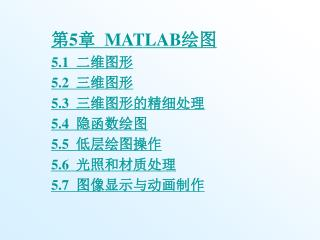DownloadDownload Presentation# 第 5 章 MATLAB 绘图 5.1 二维图形 5.2 三维图形 5.3 三维图形的精细处理 5.4 隐函数绘图 5.5 低层绘图操作 5.6 光照和材质处理

Download Presentation## 第 5 章 MATLAB 绘图 5.1 二维图形 5.2 三维图形 5.3 三维图形的精细处理 5.4 隐函数绘图 5.5 低层绘图操作 5.6 光照和材质处理

- - - - - - - - - - - - - - - - - - - - - - - - - - - E N D - - - - - - - - - - - - - - - - - - - - - - - - - - -
##### Presentation Transcript

1. 第5章 MATLAB绘图 5.1 二维图形 5.2 三维图形 5.3 三维图形的精细处理 5.4 隐函数绘图 5.5 低层绘图操作 5.6 光照和材质处理 5.7 图像显示与动画制作

2. 5.1 二维图形 5.1.1 绘制二维曲线的基本函数 1. plot函数的基本用法 plot函数的基本调用格式为： plot(x,y) 其中x和y为长度相同的向量，分别用于存储x坐标和y坐标数据。

3. 例5.1在0≤X≤2区间内，绘制曲线y=2e-0.5xsin(2πx)。例5.1在0≤X≤2区间内，绘制曲线y=2e-0.5xsin(2πx)。 程序如下： x=0:pi/100:2*pi; y=2*exp(-0.5*x).*sin(2*pi*x); plot(x,y)

4. 例5.2 绘制参数方程曲线。 程序如下： t=-pi:pi/100:pi; x=t.*cos(3*t); y=t.*sin(t).*sin(t); plot(x,y);

5. 说明： （1）当x,y是同维矩阵时，则以x,y对应列元素为横、纵坐标分别绘制曲线，曲线条数等于矩阵的列数。 （2）当x是向量，y是有一维与x同维的矩阵时，则绘制出多根不同色彩的曲线。曲线条数等于y矩阵的另一维数，x被作为这些曲线共同的横坐标。 （3）plot函数最简单的调用格式是只包含一个输入参数：plot(x)。

6. 2．含多个输入参数的plot函数 含多个输入参数的plot函数调用格式为： plot(x1,y1,x2,y2,…,xn,yn) 3．含选项的plot函数 含选项的plot函数调用格式为： plot(x1,y1,选项1,x2,y2, 选项2,…,xn,yn,选项n)

7. 例5.3 用不同线型和颜色在同一坐标内绘制曲线y=2e-0.5xsin(2πx)及其包络线。 程序如下： x=(0:pi/100:2*pi)'; y1=2*exp(-0.5*x)*[1,-1]; y2=2*exp(-0.5*x).*sin(2*pi*x); x1=(0:12)/2; y3=2*exp(-0.5*x1).*sin(2*pi*x1); plot(x,y1,'g:',x,y2,'b--',x1,y3,'rp');

8. 4．双纵坐标函数plotyy plotyy函数是MATLAB 5.X新增的函数。它能把函数值具有不同量纲、不同数量级的两个函数绘制在同一坐标中。调用格式为： plotyy(x1,y1,x2,y2) 其中x1-y1对应一条曲线，x2-y2对应另一条曲线。横坐标的标度相同，纵坐标有两个，左纵坐标用于x1-y1数据对，右纵坐标用于x2-y2数据对。

9. 例5.4用不同标度在同一坐标内绘制曲线y1=e-0.5xsin(2πx)及曲线y2=1.5e-0.1xsin(x)。例5.4用不同标度在同一坐标内绘制曲线y1=e-0.5xsin(2πx)及曲线y2=1.5e-0.1xsin(x)。 程序如下： x1=0:pi/100:2*pi; x2=0:pi/100:3*pi; y1=exp(-0.5*x1).*sin(2*pi*x1); y2=1.5*exp(-0.1*x2).*sin(x2); plotyy(x1,y1,x2,y2);

10. 5.1.2 绘制图形的辅助操作 1. 图形标注 有关图形标注函数的调用格式为： title(图形名称) xlabel(x轴说明) ylabel(y轴说明) text(x,y,图形说明) legend(图例1,图例2,…)

11. 2. 坐标控制 函数的调用格式为： axis([xmin xmax ymin ymax zmin zmax]) axis函数功能丰富，常用的用法还有： axis equal 纵、横坐标轴采用等长刻度 axis square 产生正方形坐标系(缺省为矩形) axis auto 使用缺省设置 axis off 取消坐标轴 axis on 显示坐标轴 grid on/off命令控制是画还是不画网格线，不带参数的grid命令在两种状态之间进行切换。 box on/off命令控制是加还是不加边框线，不带参数的box命令在两种状态之间进行切换。

12. 例5.5 绘制分段函数曲线并添加图形标注。 程序如下： x=linspace(0,10,100); y=[]; for x0=x if x0>=8 y=[y,1]; elseif x0>=6 y=[y,5-x0/2]; elseif x0>=4 y=[y,2]; elseif x0>=0 y=[y,sqrt(x0)]; end end plot(x,y) axis([0 10 0 2.5]) %设置坐标轴 title('分段函数曲线'); %加图形标题 xlabel('Variable X'); %加X轴说明 ylabel('Variable Y'); %加Y轴说明 text(2,1.3,'y=x^{1/2}'); %在指定位置添加图形说明 text(4.5,1.9,'y=2'); text(7.3,1.5,'y=5-x/2'); text(8.5,0.9,'y=1');

13. 3. 图形保持 一般情况下，绘图命令每执行一次就刷新当前图形窗口，图形窗口原有图形将不复存在。若希望在已存在的图形上再继续添加新的图形，可使用图形保持命令hold。hold on/off命令控制是保持原有图形还是刷新原有图形，不带参数的hold命令在两种状态之间进行切换。

14. 例5.6 用图形保持功能在同一坐标内绘制曲线y=2e-0.5xsin(2πx)及其包络线。 程序如下： x=(0:pi/100:2*pi)'; y1=2*exp(-0.5*x)*[1,-1]; y2=2*exp(-0.5*x).*sin(2*pi*x); plot(x,y1,'b:'); axis([0,2*pi,-2,2]); %设置坐标 hold on; %设置图形保持状态 plot(x,y2,'k'); legend('包络线','包络线','曲线y'); %加图例 hold off; %关闭图形保持 grid %网格线控制

15. 4. 图形窗口的分割 subplot函数的调用格式为： subplot(m,n,p) 例5.7 在一个图形窗口中以子图形式同时绘制正弦、余弦、正切、余切曲线。 程序如下： x=linspace(0,2*pi,60); y=sin(x);z=cos(x); t=sin(x)./(cos(x)+eps); ct=cos(x)./(sin(x)+eps); subplot(2,2,1); plot(x,y);title('sin(x)');axis ([0,2*pi,-1,1]); subplot(2,2,2); plot(x,z);title('cos(x)');axis ([0,2*pi,–1,1]); subplot(2,2,3); plot(x,t);title('tangent(x)');axis ([0,2*pi,-40,40]); subplot(2,2,4); plot(x,ct);title('cotangent(x)');axis ([0,2*pi,-40,40]);

16. 对图形窗口灵活分割。请看下面的程序。 x=linspace(0,2*pi,60); y=sin(x);z=cos(x); t=sin(x)./(cos(x)+eps); ct=cos(x)./(sin(x)+eps); subplot(2,2,1); %选择2×2个区中的1号区 stairs(x,y);title('sin(x)-1');axis ([0,2*pi,-1,1]); subplot(2,1,2); %选择2×1个区中的2号区 stem(x,y);title('sin(x)-2');axis ([0,2*pi,-1,1]); subplot(4,4,3); %选择4×4个区中的3号区 plot(x,y);title('sin(x)');axis ([0,2*pi,-1,1]); subplot(4,4,4); %选择4×4个区中的4号区 plot(x,z);title('cos(x)');axis ([0,2*pi,-1,1]); subplot(4,4,7); %选择4×4个区中的7号区 plot(x,t);title('tangent(x)');axis ([0,2*pi,-40,40]); subplot(4,4,8); %选择4×4个区中的8号区 plot(x,ct);title('cotangent(x)');axis ([0,2*pi,-40,40]);

17. 5.1.3 绘制二维图形的其他函数 1. 其他形式的线性直角坐标图 在线性直角坐标系中，其他形式的图形有条形图、阶梯图、杆图和填充图等，所采用的函数分别是： bar(x,y,选项) stairs(x,y,选项) stem(x,y,选项) fill(x1,y1,选项1,x2,y2,选项2,…)

18. 例5.8分别以条形图、填充图、阶梯图和杆图形式绘制曲线y=2e-0.5x。例5.8分别以条形图、填充图、阶梯图和杆图形式绘制曲线y=2e-0.5x。 程序如下： x=0:0.35:7; y=2*exp(-0.5*x); subplot(2,2,1);bar(x,y,'g'); title('bar(x,y,''g'')');axis([0,7,0,2]); subplot(2,2,2);fill(x,y,'r'); title('fill(x,y,''r'')');axis([0,7,0,2]); subplot(2,2,3);stairs(x,y,'b'); title('stairs(x,y,''b'')');axis([0,7,0,2]); subplot(2,2,4);stem(x,y,'k'); title('stem(x,y,''k'')');axis([0,7,0,2]);

19. 2．极坐标图 polar函数用来绘制极坐标图，其调用格式为： polar(theta,rho,选项) 其中theta为极坐标极角，rho为极坐标矢径，选项的内容与plot函数相似。 例5.9绘制ρ=sin(2θ)cos(2θ)的极坐标图。 程序如下： theta=0:0.01:2*pi; rho=sin(2*theta).*cos(2*theta); polar(theta,rho,'k');

20. 3．对数坐标图形 MATLAB提供了绘制对数和半对数坐标曲线的函数，调用格式为： semilogx(x1,y1,选项1,x2,y2,选项2,…) semilogy(x1,y1,选项1,x2,y2,选项2,…) loglog(x1,y1,选项1,x2,y2,选项2,…)

21. 例5.10绘制y=10x2的对数坐标图并与直角线性坐标图进行比较。例5.10绘制y=10x2的对数坐标图并与直角线性坐标图进行比较。 程序如下： x=0:0.1:10; y=10*x.*x; subplot(2,2,1);plot(x,y);title('plot(x,y)');grid on; subplot(2,2,2);semilogx(x,y);title('semilogx(x,y)'); grid on; subplot(2,2,3);semilogy(x,y);title('semilogy(x,y)'); grid on; subplot(2,2,4);loglog(x,y);title('loglog(x,y)');grid on;

22. 4. 对函数自适应采样的绘图函数 fplot函数的调用格式为： fplot(fname,lims,tol,选项) 例5.11 用fplot函数绘制f(x)=cos(tan(πx))的曲线。 先建立函数文件myf.m： function y=myf(x) y=cos(tan(pi*x)); 再用fplot函数绘制myf.m函数的曲线： fplot('myf',[-0.4,1.4],1e-4)

23. 5. 其他形式的图形 MATLAB提供的绘图函数还有很多，例如，用来表示各元素占总和的百分比的饼图、复数的相量图等等。 例5.12绘制图形： (1)某次考试优秀、良好、中等、及格、不及格的人数分别为：7,17,23,19,5，试用饼图作成绩统计分析。 (2)绘制复数的相量图：3+2i、4.5-i和-1.5+5i。 程序如下： subplot(1,2,1); pie([7,17,23,19,5]); title('饼图');legend('优秀','良好','中等','及格','不及格'); subplot(1,2,2); compass([3+2i,4.5-i,-1.5+5i]);title('相量图');

24. 5.2 三维图形 5.2.1 绘制三维曲线的基本函数 plot3函数与plot函数用法十分相似，其调用格式为： plot3(x1,y1,z1,选项1,x2,y2,z2,选项2,…,xn,yn,zn,选项n) 例5.13 绘制空间曲线。 程序如下： t=0:pi/50:2*pi; x=8*cos(t); y=4*sqrt(2)*sin(t); z=-4*sqrt(2)*sin(t); plot3(x,y,z,'p'); title('Line in 3-D Space');text(0,0,0,'origin'); xlabel('X'),ylabel('Y'),zlabel('Z');grid;

25. 5.2.2 三维曲面 1．平面网格坐标矩阵的生成 (1)利用矩阵运算生成。 x=a:dx:b; y=(c:dy:d)'; X=ones(size(y))*x; Y=y*ones(size(x)); (2)利用meshgrid函数生成。 x=a:dx:b; y=c:dy:d; [X,Y]=meshgrid(x,y);

26. 例5.14 已知6<x<30，15<y<36，求不定方程2x+5y=126的整数解。 程序如下： x=7:29; y=16:35; [x,y]=meshgrid(x,y); %在[5,29]×[14,35]区域生成网格坐标 z=2*x+5*y; k=find(z==126); %找出解的位置 x(k),y(k) %输出对应位置的x,y即方程的解

27. 2. 绘制三维曲面的函数 surf函数和mesh函数的调用格式为： mesh(x,y,z,c) surf(x,y,z,c) 例5.15用三维曲面图表现函数z=sin(y)cos(x)。 程序1： x=0:0.1:2*pi;[x,y]=meshgrid(x);z=sin(y).*cos(x); mesh(x,y,z);xlabel('x-axis'),ylabel('y-axis'),zlabel('z-axis');title('mesh'); 程序2： x=0:0.1:2*pi;[x,y]=meshgrid(x);z=sin(y).*cos(x); surf(x,y,z);xlabel('x-axis'),ylabel('y-axis'),zlabel('z-axis');title('surf'); 程序3： x=0:0.1:2*pi;[x,y]=meshgrid(x);z=sin(y).*cos(x); plot3(x,y,z);xlabel('x-axis'),ylabel('y-axis'),zlabel('z-axis');title('plot3-1');grid;

28. 例5.16 绘制两个直径相等的圆管的相交图形。 程序如下: %两个等直径圆管的交线 m=30; z=1.2*(0:m)/m; r=ones(size(z)); theta=(0:m)/m*2*pi; x1=r'*cos(theta);y1=r'*sin(theta); %生成第一个圆管的坐标矩阵 z1=z'*ones(1,m+1); x=(-m:2:m)/m; x2=x'*ones(1,m+1);y2=r'*cos(theta); %生成第二个圆管的坐标矩阵 z2=r'*sin(theta); surf(x1,y1,z1); %绘制竖立的圆管 axis equal,axis off hold on surf(x2,y2,z2); %绘制平放的圆管 axis equal,axis off title('两个等直径圆管的交线'); hold off

29. 例5.17分析由函数z=x2-2y2构成的曲面形状及与平面z=a的交线。例5.17分析由函数z=x2-2y2构成的曲面形状及与平面z=a的交线。 程序如下： [x,y]=meshgrid(-10:0.2:10); z1=(x.^2-2*y.^2)+eps; %第1个曲面 a=input('a=?'); z2=a*ones(size(x)); %第2个曲面 subplot(1,2,1);mesh(x,y,z1);hold on;mesh(x,y,z2); %分别画出两个曲面 v=[-10,10,-10,10,-100,100];axis(v);grid; %第1子图的坐标设置 hold off; r0=abs(z1-z2)<=1; %求两曲面z坐标差小于1的点 xx=r0.*x; yy=r0.*y; zz=r0.*z2; %求这些点上的x,y,z坐标，即交线坐标 subplot(1,2,2); plot3(xx(r0~=0),yy(r0~=0),zz(r0~=0),'*'); %在第2子图画出交线 axis(v);grid; %第2子图的坐标设置

30. 例5.18 在xy平面内选择区域[-8,8]×[-8,8]，绘制函数的4种三维曲面图。 程序如下： [x,y]=meshgrid(-8:0.5:8); z=sin(sqrt(x.^2+y.^2))./sqrt(x.^2+y.^2+eps); subplot(2,2,1); meshc(x,y,z); title('meshc(x,y,z)') subplot(2,2,2); meshz(x,y,z); title('meshz(x,y,z)') subplot(2,2,3); surfc(x,y,z) title('surfc(x,y,z)') subplot(2,2,4); surfl(x,y,z) title('surfl(x,y,z)')

31. 3. 标准三维曲面 • sphere函数的调用格式为： [x,y,z]=sphere(n) • cylinder函数的调用格式为： [x,y,z]=sphere(R,n) • MATLAB还有一个peaks 函数，称为多峰函数，常用于三维曲面的演示。

32. 例5.19 绘制标准三维曲面图形。 程序如下： t=0:pi/20:2*pi; [x,y,z]= cylinder(2+sin(t),30); subplot(1,3,1); surf(x,y,z); subplot(1,3,2); [x,y,z]=sphere; surf(x,y,z); subplot(1,3,3); [x,y,z]=peaks(30); meshz(x,y,z);

33. 5.2.3 其他三维图形 条形图、饼图和填充图等特殊图形，它们还可以以三维形式出现，使用的函数分别是bar3、pie3和fill3。此外，还有三维曲面的等高线图。等高线图分二维和三维两种形式，分别使用函数contour和contour3绘制。

34. 例5.20 绘制三维图形： (1)绘制魔方阵的三维条形图。 (2)以三维杆图形式绘制曲线y=2sin(x)。 (3)已知x=[2347,1827,2043,3025]，绘制三维饼图。 (4)用随机的顶点坐标值画出五个黄色三角形。 程序如下： subplot(2,2,1); bar3(magic(4)) subplot(2,2,2); y=2*sin(0:pi/10:2*pi); stem3(y); subplot(2,2,3); pie3([2347,1827,2043,3025]); subplot(2,2,4); fill3(rand(3,5),rand(3,5),rand(3,5), 'y' )

35. 例5.21 绘制多峰函数的瀑布图和等高线图。 程序如下： subplot(1,2,1); [X,Y,Z]=peaks(30); waterfall(X,Y,Z) xlabel('X-axis'),ylabel('Y-axis'),zlabel('Z-axis'); subplot(1,2,2); contour3(X,Y,Z,12,'k'); %其中12代表高度的等级数 xlabel('X-axis'),ylabel('Y-axis'),zlabel('Z-axis');

36. 5.3 三维图形的精细处理 5.3.1 视点处理 MATLAB提供了设置视点的函数view。其调用格式为： view(az,el) 其中az为方位角，el为仰角，它们均以度为单位。系统缺省的视点定义为方位角-37.5°,仰角30°。

37. 例5.22 从不同视点绘制多峰函数曲面。 程序如下： subplot(2,2,1);mesh(peaks); view(-37.5,30); %指定子图1的视点 title('azimuth=-37.5,elevation=30') subplot(2,2,2);mesh(peaks); view(0,90); %指定子图2的视点 title('azimuth=0,elevation=90') subplot(2,2,3);mesh(peaks); view(90,0); %指定子图3的视点 title('azimuth=90,elevation=0') subplot(2,2,4);mesh(peaks); view(-7,-10); %指定子图4的视点 title('azimuth=-7,elevation=-10')

38. 5.3.2 色彩处理 1. 颜色的向量表示 MATLAB除用字符表示颜色外，还可以用含有3个元素的向量表示颜色。 2. 色图 色图是m×3 的数值矩阵，它的每一行是RGB三元组。色图矩阵可以人为地生成，也可以调用MATLAB提供的函数来定义色图矩阵。 除plot及其派生函数外，mesh、surf等函数均使用色图着色。图形窗口色图的设置和改变，使用函数： colormap(m) 其中m代表色图矩阵。 3. 三维表面图形的着色 三维表面图实际上就是在网格图的每一个网格片上涂上颜色。surf函数用缺省的着色方式对网格片着色。除此之外，还可以用shading命令来改变着色方式。

40. 5.3.3 图形的裁剪处理 MATLAB定义的NaN常数可以用于表示那些不可使用的数据，利用这种特性，可以将图形中需要裁剪部分对应的函数值设置成NaN，这样在绘制图形时，函数值为NaN的部分将不显示出来，从而达到对图形进行裁剪的目的。 例如，要削掉正弦波顶部或底部大于0.5的部分，可使用下面的程序： x=0:pi/10:4*pi; y=sin(x); i=find(abs(y)>0.5); x(i)=NaN; plot(x,y);

41. 例5.24 绘制两个球面，其中一个球在另一个球里面，将外面的球裁掉一部分，使得能看见里面的球。 程序如下： [x,y,z]=sphere(20); %生成外面的大球 z1=z; z1(:,1:4)=NaN; %将大球裁掉一部分 c1=ones(size(z1)); surf(3*x,3*y,3*z1,c1); %生成里面的小球 hold on z2=z; c2=2*ones(size(z2)); c2(:,1:4)=3*ones(size(c2(:,1:4))); surf(1.5*x,1.5*y,1.5*z2,c2); colormap([0,1,0;0.5,0,0;1,0,0]); grid on hold off

42. 5.4 隐函数绘图 MATLAB提供了一个ezplot函数绘制隐函数图形，下面介绍其用法。 (1) 对于函数f = f(x)，ezplot函数的调用格式为： ezplot(f)：在默认区间-2π<x<2π绘制f = f(x)的图形。 ezplot(f, [a,b])：在区间a<x<b绘制f = f(x)的图形。

43. (2) 对于隐函数f = f(x,y)，ezplot函数的调用格式为： ezplot(f)：在默认区间-2π<x<2π和-2π<y<2π绘制f(x,y) = 0的图形。 ezplot(f, [xmin,xmax,ymin,ymax])：在区间xmin<x<xmax和ymin<y<ymax绘制f(x,y) = 0的图形。 ezplot(f, [a,b])：在区间a<x<b和a<y< b绘制f(x,y) = 0的图形。

44. (3) 对于参数方程x = x(t)和y = y(t)，ezplot函数的调用格式为： ezplot(x,y)：在默认区间0<t<2π绘制x=x(t)和y=y(t)的图形。 ezplot(x,y, [tmin,tmax])：在区间tmin < t < tmax绘制x=x(t)和y=y(t)的图形。

45. 例5.25 隐函数绘图应用举例。 程序如下： subplot(2,2,1); ezplot('x^2+y^2-9');axis equal subplot(2,2,2); ezplot('x^3+y^3-5*x*y+1/5') subplot(2,2,3); ezplot('cos(tan(pi*x))',[ 0,1]) subplot(2,2,4); ezplot('8*cos(t)','4*sqrt(2)*sin(t)',[0,2*pi])

46. 5.5 低层绘图操作 5.5.1 图形对象及其句柄 1. 图形对象 MATLAB把构成图形的各个基本要素称为图形对象。这些对象包括计算机屏幕、图形窗口(Figure)、坐标轴(Axes)、用户菜单(Uimenu)、用户控件(Uicontrol)、曲线(Line)、曲面(Surface)、文字(Text)、图像(image)、光源(Light)、区域块(Patch)和方框(Rectangle)。系统将每一个对象按树型结构组织起来。每个图形对象都可以被独立地操作。

47. 2. 图形对象句柄 MATLAB在创建每一个图形对象时，都为该对象分配唯一的一个值，称其为图形对象句柄(Handle)。句柄是图形对象的唯一标识符。 MATLAB提供了3个用于获取已有图形对象句柄的函数： gcf 获取当前图形窗口的句柄(get current figure)。 gca 获取当前坐标轴的句柄(get current axis)。 gco 获取最近被单击的图形对象的句柄(get current object)。

48. 5.5.2 图形对象属性 1. 属性名与属性值 MATLAB给每种对象的每一个属性规定了一个名字，称为属性名，而属性名的取值成为属性值。例如，LineStyle是曲线对象的一个属性名，它的值决定着线型，取值可以是'-' 、':'、'-.'、'--'或'none'。 2. 属性的操作 set函数的调用格式为： set(句柄，属性名1，属性值1，属性名2，属性值2，…) get函数的调用格式为： V=get(句柄，属性名)

49. 3. 对象的公共属性 图形对象具有各种各样的属性，有些属性是所有对象共同具备的，有些则是各对象所特有的。 例5.26 在同一坐标下画红、绿两根不同曲线，希望获得绿色曲线的句柄，并对其进行设置。 程序如下： x=0:pi/50:2*pi; y=sin(x); z=cos(x); plot(x,y,'r',x,z,'g'); H=get(gca,'Children'); %获取两曲线句柄向量H for k=1:length(H) if get(H(k),'Color')==[0 1 0] %[0 1 0]代表绿色 Hg=H(k); %获取绿色线条句柄 end end pause %便于观察设置效果 set(Hg,'LineStyle',':','Marker','p'); %对绿色曲线进行设置

50. 5.5.3 图形对象的创建 1. 图形窗口对象 建立图形窗口对象使用figure函数。调用该函数的命令形式为： 句柄变量=figure(属性名1，属性值1，属性名2，属性值2，…) MATLAB为每个图形窗口提供了很多属性。这些属性及其取值控制着图形窗口对象。除公共属性外，其他常用属性：MenuBar属性、Name属性、Position属性、Color属性等。